Algebra of continous functions

Chapter 5 Class 12 Continuity and Differentiability
Concept wise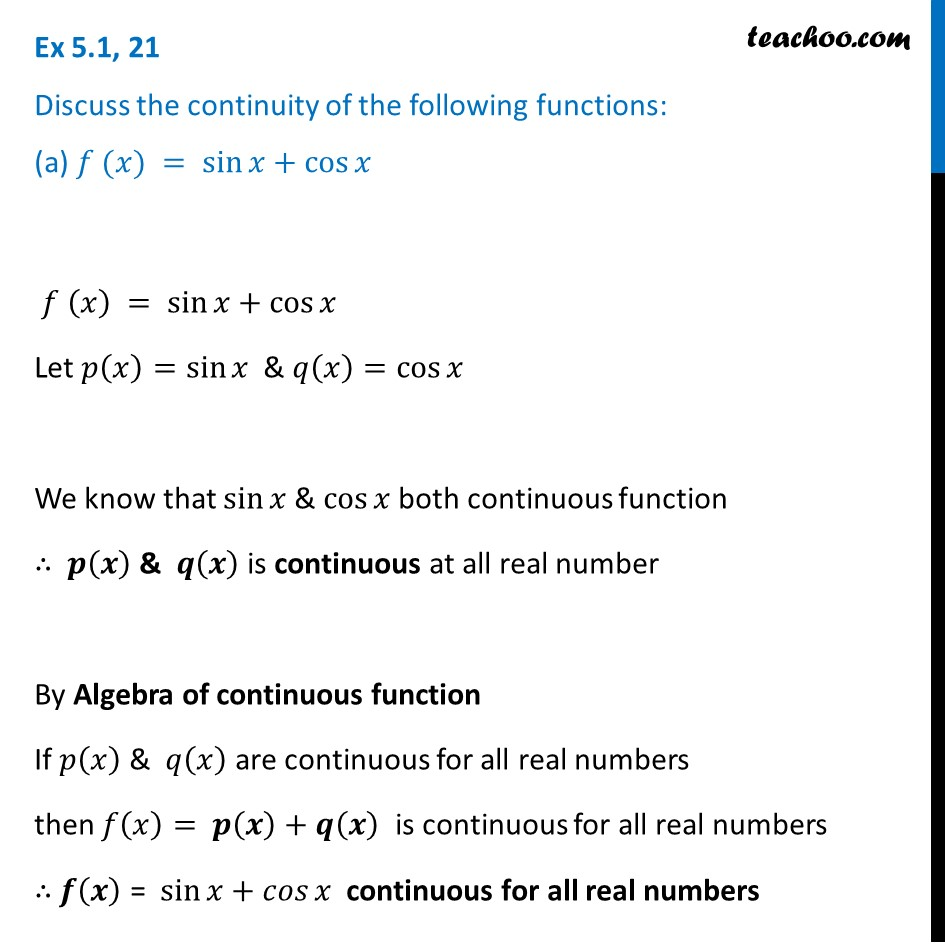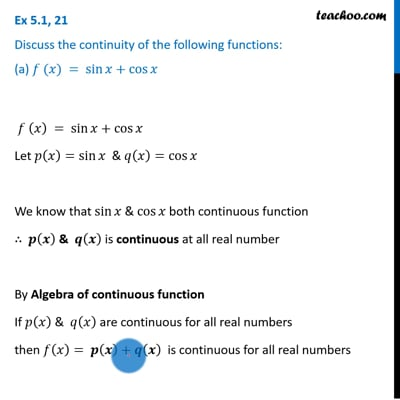This video is only available for Teachoo black users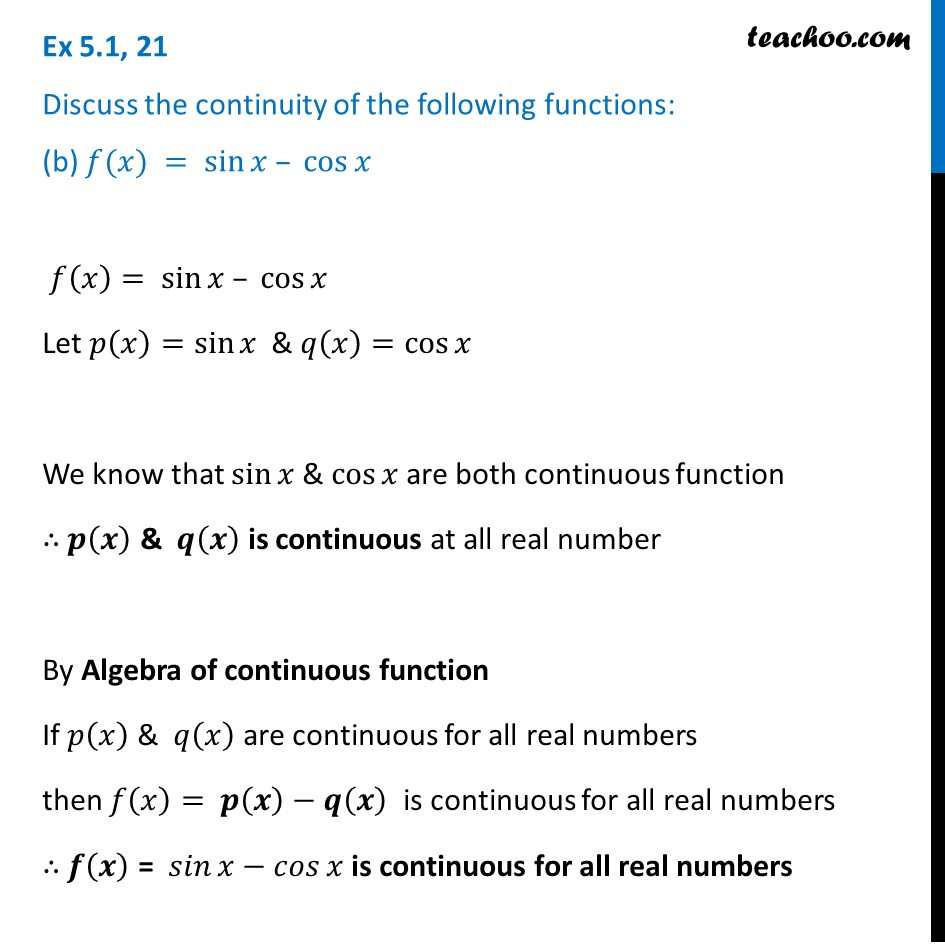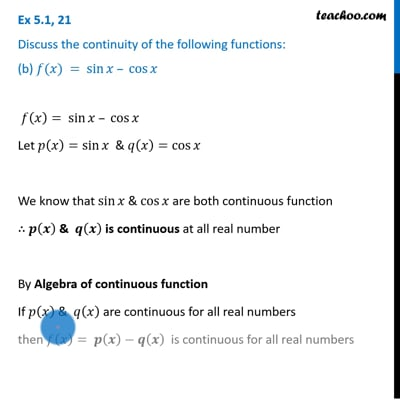This video is only available for Teachoo black users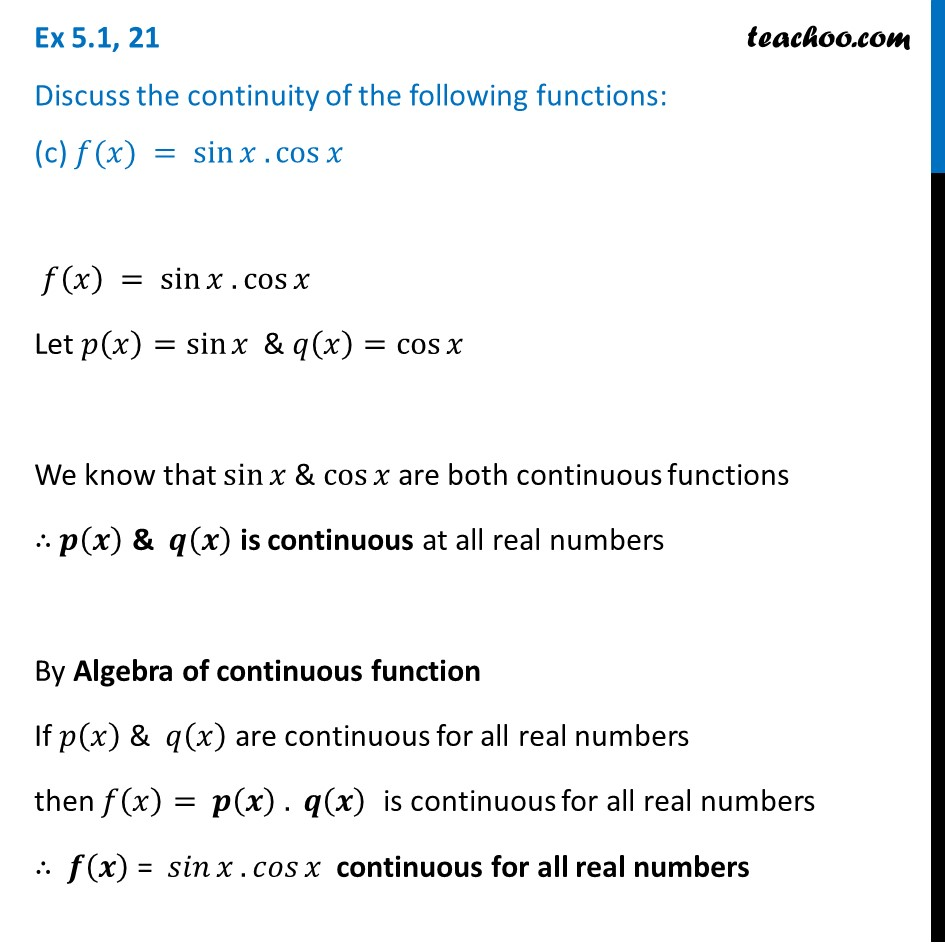Introducing your new favourite teacher - Teachoo Black, at only ₹83 per month

### Transcript

Ex 5.1, 21 Discuss the continuity of the following functions: (a) 𝑓 (𝑥) = sin⁡𝑥+cos⁡𝑥 𝑓 (𝑥) = sin⁡𝑥+cos⁡𝑥 Let 𝑝(𝑥)=sin⁡𝑥 & 𝑞(𝑥)=cos⁡𝑥" " We know that sin⁡𝑥 & cos⁡𝑥 both continuous function ∴ 𝒑(𝒙) & 𝒒(𝒙) is continuous at all real number By Algebra of continuous function If 𝑝(𝑥)" & " 𝑞(𝑥) are continuous for all real numbers then 𝑓(𝑥)= 𝒑(𝒙)+𝒒(𝒙) is continuous for all real numbers ∴ 𝒇(𝒙) = sin⁡𝑥+𝑐𝑜𝑠⁡𝑥 continuous for all real numbers Ex 5.1, 21 Discuss the continuity of the following functions: (b) 𝑓(𝑥) = sin⁡𝑥 – cos⁡𝑥 𝑓(𝑥)= sin⁡𝑥 – cos⁡𝑥 Let 𝑝(𝑥)=sin⁡𝑥 & 𝑞(𝑥)=cos⁡𝑥" " We know that sin⁡𝑥 & cos⁡𝑥 are both continuous function ∴ 𝒑(𝒙) & 𝒒(𝒙) is continuous at all real number By Algebra of continuous function If 𝑝(𝑥)" & " 𝑞(𝑥) are continuous for all real numbers then 𝑓(𝑥)= 𝒑(𝒙)−𝒒(𝒙) is continuous for all real numbers ∴ 𝒇(𝒙) = 𝑠𝑖𝑛⁡𝑥−𝑐𝑜𝑠⁡𝑥 is continuous for all real numbers Ex 5.1, 21 Discuss the continuity of the following functions: (c) 𝑓(𝑥) = sin⁡𝑥 . cos⁡𝑥 𝑓(𝑥) = sin⁡𝑥 . cos⁡𝑥 Let 𝑝(𝑥)=sin⁡𝑥 & 𝑞(𝑥)=cos⁡𝑥" " We know that sin⁡𝑥 & cos⁡𝑥 are both continuous functions ∴ 𝒑(𝒙) & 𝒒(𝒙) is continuous at all real numbers By Algebra of continuous function If 𝑝(𝑥)" & " 𝑞(𝑥) are continuous for all real numbers then 𝑓(𝑥)= 𝒑(𝒙) . 𝒒(𝒙) is continuous for all real numbers ∴ 𝒇(𝒙) = 𝑠𝑖𝑛⁡𝑥.𝑐𝑜𝑠⁡𝑥 continuous for all real numbers